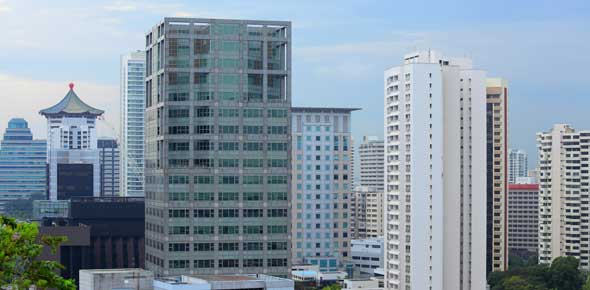# Masonry And Concrete

10 Questions | Total Attempts: 1070Settings• 1.
The most widely used masonry materials for construction works.
• A.

A. concrete hollow blocks

• B.

B. bricks

• C.

C. rubble stones

• D.

D. ashlar

• 2.
it is the masonry material manufactured from clay and other materials.
• A.

A. stones

• B.

• C.

C. bricks

• D.

D. course rubble's

• 3.
What is the tool used to guide the vertical position in laying bricks?
• A.

A. plumb bob

• B.

B. water hose

• C.

C. spirit level

• D.

D. spring or chord

• 4.
Which of the following is NOT a requirement to consider in proportioning concrete mixture?
• A.

A. economy

• B.

B. workability

• C.

C. strength

• D.

D. flexibility

• 5.
Concrete is said to be workable if it can be molded or deformed without segregation. this characteristics is known as___.
• A.

A. consistency

• B.

B. plasticity

• C.

C. mobility

• D.

D. workability

• 6.
Which of t he following classes of mixture produces a hard or tough concrete?
• A.

A. class AA

• B.

B. class A

• C.

C. class B

• D.

D. class C

• 7.
A good concrete can be produced also by hand mixing. which of the following is a good concrete mix proportion for hand mixing?
• A.

A. 1/2 kg of water + 1 kg of cement + 2 kg sand + 2.5 to 3 kg of gravel

• B.

B. 2 kg of water + 1 kg of cement + 2 kg sand + 2.5 to 3 kg of gravel

• C.

C. 1/2 kg of water + 2 kg of cement + 2 kg sand + 2.5 to 3 kg of gravel

• D.

D. 1/2 kg of water + 2 kg of cement + 3 kg sand + 2.5 to 3 kg of gravel

• 8.
Which of the following should be avoided in placing concrete to its final form?
• A.

A. segregation of particles

• B.

B. displacement of form and displacement of reinforcement in the form

• C.

C. poor bond between successive layers of concrete

• D.

D. all of the above should be avoided

• 9.
Gravel tends to settle, lighter materials and water tend to rise inside a container when delayed in the delivery to the form. what do you call this tendency?
• A.

A. displacement proportion of the ingredients

• B.

B. segregation of particles

• C.

C. consistency

• D.

D. mobility of paticles

• 10.
Which factor/s regulates the strenght of concrete?
• A.

A. correct proportion of the ingredients

• B.

B. proper method of mixing

• C.

C. adequate protection of concrete during the process of curing

• D.

D. all of the above mentioned factors

Related TopicsBack to top